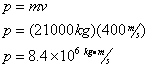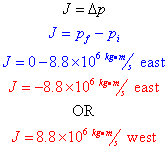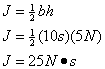# Momentum & Impulse

### Defining Momentum

Let’s assume there’s a car speeding toward you, out of control without its brakes, at a speed of 27 m/s (60 mph). Can you stop it by standing in front of it and holding out your hand? Why not?Unless you're Superman, you probably don't want to try stopping a moving car by holding out your hand. It's too big, and it's moving way too fast. Attempting such a feat would result in a number of physics demonstrations upon your body, all of which would hurt.

We can't stop the car because it has too much momentum. Momentum is a vector quantity, given the symbol p, which measures how hard it is to stop a moving object. Of course, larger objects have more momentum than smaller objects, and faster objects have more momentum than slower objects. We can therefore calculate momentum using the equation:Momentum is the product of an object's mass times its velocity, and its units must be the same as the units of mass [kg] times velocity [m/s], therefore the units of momentum must be [kg*m/s], which can also be written as a Newton-second [N*s].

Question: Two trains, Big Red and Little Blue, have the same velocity. Big Red, however, has twice the mass of Little Blue. Compare their momenta.

Answer: Because Big Red has twice the mass of Little Blue, and Big Red must have twice the momentum of Little Blue.

Because momentum is a vector, the direction of the momentum vector is the same as the direction of the object's velocity.Question: An A-5 Vigilante supersonic bomber, with a mass of 21000 kg, departs from its home airbase with a velocity of 400 m/s due east. What is the jet's momentum?

Answer:Now, let's assume the jet drops its payload and has burned up most of its fuel as it continues its journey to its destination air field.

Question: If the jet's new mass is 16,000 kg, and due to its reduced weight the pilot increases the cruising speed to 550 m/s, what is the jet's new momentum?

Answer:### Impulse

As you can see, momentum can change, and a change in momentum is known as an impulse. In Regents Physics, the vector quantity impulse is represented by a capital J, and since it's a change in momentum, its units are the same as those for momentum, [kg*m/s], and can also be written as a Newton-second [N*s].Question: Assume the A-5 bomber, which had a momentum of 8.8*106 kg*m/s, comes to a halt on the ground. What impulse is applied?

Answer: Let's assume that east is the positive direction:### Impulse-Momentum Theorem

Since momentum is equal to mass times velocity, we can write that. We also know that impulse is a change in momentum, so impulse can be written as. If we combine these equations, we find:Since the mass of a single object is constant, a change in the product of mass and velocity is equivalent to the product of mass and change in velocity. Specifically:So we're talking about changes in velocity... but what do we call changes in velocity? Of course, acceleration! And what causes an acceleration? A force! And does it matter if the force is applied for a very short time or a very long time? Absolutely it does -- common sense tells us the longer the force is applied, the longer the object will accelerate, the greater the object's change in momentum!

We can prove this by using an old mathematician's trick -- if we multiply the right side of our equation by 1, we of course get the same thing. And if we multiply the right side of our equation by Δt/Δt, which is 1, we still get the same thing. Take a look:If you look carefully at this equation, you can find a Δv/Δt, which is, by definition acceleration. Let's replace Δv/Δt with acceleration a in the equation below.One last step... perhaps you can see it already. On the right-hand side of this equation, we have maΔt. Utilizing Newton's 2nd Law, I can replace the product of mass and acceleration with force F, giving us the final form of our equation, often-times referred to as the Impulse-Momentum Theorem:This equation relates impulse to change in momentum to force applied over a time interval.

To summarize: When an unbalanced force acts on an object for a period of time, a change in momentum is produced, known as an impulse.Question: A tow-truck applies a force of 2000N on a 2000-kg car for a period of 3 seconds. What is the magnitude of the change in the car's momentum?

Answer:Question: If the car starts at rest, what will be its speed after 3s?

Answer:Let's have you try one on your own...

### Non-Constant ForcesBut not all forces are constant... what do you do if a changing force is applied for a period of time? In that case, we can make a graph of the force applied on the y-axis vs. time on the x-axis. The area under the Force-Time curve is the impulse, or change in momentum.

For the case of the sample graph at the right, we could determine the impulse applied by calculating the area of the triangle under the curve. In this case: